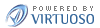Compare Datasets Here you have the possibility to compare two different datasets. The line on top represents the first dataset, the line below the second one.

Model selection
Please choose a model and than one of its datasets.

Dataset selection

Overall numbers:
A short summary of numbers counting important elements.0
Dimensions0
Dimension-
elements0
Measures0
Attributes0
Slices0
Observations

Measure information

 Range Median Mean Variance Standard Deviation It describes the lowest and highest values of all observated values. The numerical value separating the higher half from the lower half. It is the average value. The variance is a measure of how far a list of observated values is spread out. It shows how much variation exists from the average (mean), or expected value. 0 0 0 0 0 0
Invalid numbers occour in the observations, so computed values are not exact!
Unequal dimensions

Equal dimensions

For comparision you have to choose another model and one of its datasets.0
Dimensions0
Dimension-
elements0
Measures0
Attributes0
Slices0
Observations

 Range Median Mean Variance Standard Deviation 0 0 0 0 0 0
Invalid numbers occour in the observations, so computed values are not exact!
No equal dimensions found! Please selected another dataset or enrich datasets with relations to each other.

Now i provide you a couple of different ways to explore and compare the data you selected before.

Configure data for further exploration

In this area you can adapt the observations or their meta-data.

Normalization of observation values

Dataset 1

Dataset 2

 Placeholders \$value\$ represents the value of a observation \$pi\$ conforms to π
 Basic operators + - * /
 Functions log (x) The natural logarithm for x sqrt(x) It will returns the square root of x sin(x) Sine of x cos(x) Cosine of x tan(x) Tangent of x asin(x) Arcsine of x acos(x) Arccosine of x atan(x) Arctangent of x

Set Dimension Elements

 Use all Observations, no matter if they are according to equal or unequal Dimensions and Dimension Elements. Only use Observations which are according to equal Dimensions with equal Dimension Elements. Only use Observations which are according to equal Dimensions with unequal Dimension Elements.

Show clusters

Maximum Number of Clusters:

Wanna explore clusters in the data? Set the number of clusters and you will get a Circle Packing visualization containing grouped observations by according cluster.